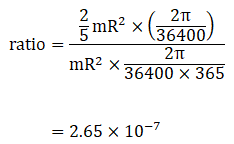# HC Verma Solutions Class 11 Chapter 10 Rotational Mechanics

HC Verma Class 11 Solutions Chapter 10 – Rotational Mechanics strives to convey the right knowledge to students about questions related to the chapter. The solutions consist of answers for the different questions present in the chapter. The problems are mostly based on finding the angular velocity of an object by referring to the graph that is provided and also finding the total rotational angle. The problems given in chapter 10 of HC Verma book can be quite challenging for the students. Therefore the solutions provided here have been prepared by experts to increase the understanding and enhance the problem-solving skills of the students. They will get to learn topics of rotational mechanics in the problems given as:

• Questions in chapter 10 are based on finding the linear velocity of the rim and at the middle of the radius.
• There are questions on finding Radial Acceleration and tangential acceleration, the axis of rotation, torque and net moment of inertia of a point in a triangle.
• Questions are also set on concepts of finding the moment of inertia at the centre of a circle and there are questions related to perpendicular to the plane of the ring along with finding the deceleration of a flywheel experiencing friction.

With such topics, we are offering HC Verma Class 11 Solutions  for class 11 students and it has been designed to help students develop the right skills to solve conceptual problems in chapter 10 and also perform well in the exams.

Some of the important topics that students will learn about in chapter 10 are;

1. Rotation of a Rigid Body about a Given Fixed Line
2. Kinematics
3. Rotational Dynamics
4. Torque of a Force about the Axis of Rotation
5. Bodies in Equilibrium
6. Bending of a Cyclist on a Horizontal Turn
7. Angular Momentum
8. Conservation of Angular Momentum
9. Angular Impulse
10. Kinetic Energy of a Rigid Body Rotating About a Given Axis
11. Power Delivered and Work Done by a Torque
12. Calculation of Moment of Inertia
13. Two Important Theorems on Moment of Inertia
14. Combined Rotation and Translation
15. Rolling
16. Kinetic Energy of a Body in Combined Rotation and Translation
17. Angular Momentum of a Body in Combined Rotation and Translation
18. Why Does a Rolling Sphere Slow Down?

## Class 11 Important Questions in Chapter 10

1. Consider the following statement, “The torque of the weight of a body about a vertical axis is zero”. Is the statement correct? Give reasons for your answer.

2. When a sphere rolls on a horizontal surface do you think that a  certain point of the sphere experiences a vertical velocity?

3. If a particle moves with a constant velocity parallel to the X-axis then its angular momentum with respect to the origin will;

(a) increase (b) decrease (c) zero (d) remains constant.

4. You are sitting firmly over a rotating stool and your arms are fully stretched. If you fold your arms then what will happen to the angular momentum about the axis of rotation.

(a) It will increase (b) decrease (c) nothing happens (d) doubles.

5. Take, for instance, there is no external force acting on a nonrigid body. Now, which of the given quantities will remain constant?

(a) kinetic energy (b) moment of inertia (c) angular momentum (d) linear momentum.

## HC Verma Solutions Vol 1 Rotational Mechanics Chapter 10

Question 1: A wheel is making revolutions about its axis with uniform angular acceleration. Starting from rest, it reaches 100 rev/sec in 4 seconds. Find the angular acceleration. Find the angle rotated during these four seconds.

Solution:

we are given that, ω0 = 0,

Final angular velocity, ω’ =100 revs/s .

t = time = 4 s.

Let α be angular acceleration.

Now, ω’ = ω0 + αt

Where ω_0 is initial angular velocity

or α = 25 rev/s2

Again, from second equation of kinematics.

θ = ω0 + (1/2) αt2

[angle rotated during 4 seconds, so t = 4 sec]

Question 2: A wheel rotating with uniform angular acceleration covers 50 revolutions in the first five seconds after the start. Find the angular acceleration and the angular velocity at the end of five seconds.

Solution:

Given: θ = 50

time = t = 5 sec.

By equation of kinematics,

θ = ωt + (1/2) α t2

or α = 4 rev/s2

Let After 5 second angular velocity will be ω’.

ω’ = ω + αt

=> ω = 20 rev/s

Question 3: A wheel starting from rest is uniformly accelerated at 4 rad/s2 for 10 seconds. It is allowed to rotate uniformly for the next 10 seconds and is finally brought to rest in the next 10 seconds. Find the total angle rotated by the wheel.

Solution:

Time duration = t =10sec.

angle rotation = θ

Maximum angular velocity = 4 x 10 = 40 rad/s

Area under the curve will decide the total angle rotated.

Area under the curve = (1/2)x10x40 + 40×10 + (1/2)x40x10 = 800 rad = total angle rotated.

Question 4: A body rotates about a fixed axis with an angular acceleration of one radian/second/ second. Through what angle does it rotate during the time in which its angular velocity increases from 5 rad/s to 15 rad/s.

Solution:

rom first equation of kinematics-

ω = ω0 + α t …(1)

Where Initial angular velocity= ω0 = 5 rad/s and Final angular velocity = ω = 15 rad/s and α = 1 rad/s2

(1) => t = 10 sec

Again,from second equation of kinematics.

θ = ω0 t + (1/2) α t2

Here θ is the total angle rotated

Question 5: Find the angular velocity of a body rotating with an acceleration of 2 rev/s2 as it completes the 5th revolution after the start.

Solution:

α = 2 rev/s2, θ = 5 rev, ωo = 0 and ω = ?

Change in angular velocity

ω2 = 2θα …(1)

=> ω = 2√5 rev/s

or θ = 10π rad and

then (1)=> ω = 2√5 rev/s

Question 6: A disc of radius 10 cm is rotating about its axis at an angular speed of 20 rad/s, Find the linear speed of

(a) a point on the rim,

(b) the middle point of a radius.

Solution:

Radius of disc = 10 cm = 0.1 m and Angular velocity = 20 rad/s

(a) linear velocity of the rim = ωr = 20×0.1 = 2 m/s

(b) Linear velocity at the middle of radius

ωr/2 = (20×0.1)/2 = 1 m/s

Question 7: A disc rotates about its axis with a constant angular acceleration of 4 rad/s2. Find the radial and tangential accelerations of a particle at a distance of 1 cm from the axis at the end of the first second after the disc starts rotating.

Solution:

t = 1 sec and r = 1 cm = 0.01 m

Tangential acceleration:

aT = r x a = 0.01 x 4 rad/s2

Angular velocity : ω = α t = 1 x 4 = 4 rad/s

Radial acceleration: ar = ω2 x r = 0.16 m/s2 or 16 cm/s2

Question 8: A block hangs from a string wrapped on a disc of radius 20 cm free to rotate about its axis which is fixed in a horizontal position. If the angular speed of the disc is 10 rad/s at some instant, with what speed is the block going down at that instant?

Solution:

Relation between angular speed and linear speed

v = r x ω

Where, Angular speed of the disc = ω = 10 rad/s and Radius of the disc = r = 20 cm or 0.20 m

v = 10 x 0.20 = 2 m/s

Question 9: Three particles, each of mass 200 g, are kept at the corners of an equilateral triangle of side 10 cm. Find the moment of inertia of the system about an axis

(a) joining two of the particles and

(b) passing through one of the particles and perpendicular to the plane of the particles.

Solution: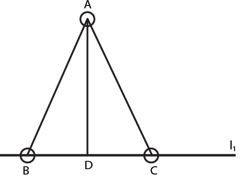The perpendicular distance from the axis AD = √3/2 x 10 = 5√3 cm

Moment of inertia about BC = l = mr2

=200 K (5√3)2 gm-cm2

= 1.5 x 10-3 kg-m2

(b) Let’s take AD as perpendicular side.

moment of inertia along BC

l = 2mr2

= 2 x 200 x 102 gm-cm2

= 4 x 10-3 kg-m2

Question 10: Particles of masses 1 g, 2 g, 3 g, …, 100 g are kept at the marks 1 cm, 2 cm, 3 cm, …, 100 cm respectively on a meter scale. Find the moment of inertia of the system of particles about a perpendicular bisector of the metre scale.

Solution: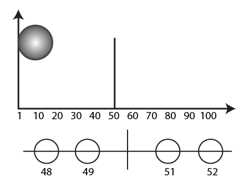Consider the two particles at the position 49 cm and 51 cm

Moment of inertia due to these 2 particles = 49×12 + 51 + 12 = 100 gm-cm2

Similarly, if consider 48th and 52nd term, we have 100 x 22 gm-cm2

Thus, we will get 49 such set and one alone partcle at 100 cm.

Total Moment of inertia = 100(12 + 22 + ….+ 492) + 100(50) 2

= 4292500 gm-cm2

= 0.43 kg-m2

Question 11: Find the moment of inertia of a pair of spheres, each having a mass m and radius r, kept in contact about the tangent passing through the point of contact.

Solution:

Moment of inertia of the first body and the 2nd body about the respective tangents becomes

MI1 = mr2 + (2/5) mr2 and MI2 = mr2 + (2/5) mr2 = (7/5) mr2

Net moment of inertia is:

MInet = (7/5) mr2 + (7/5) mr2 = (14/5) mr2 units

Question 12: The moment of inertia of a uniform rod of mass 0.50 kg and length 1 m is 0.10 kg-m2 about a line perpendicular to the rod. Find the distance of this line from the middle point of the rod.

Solution:

Length of the rod =L= 1 m, and its mass = 0.5 kg

Let r = distance between the parallel axes.

Let at a distance x from the center the rod is moving

IB = IA + IA. r2

[parallel axis theorem]

the moment of inertial at d

(mL2/12) + md2 = 0.10

On Putting values, we have

d = 0.342 m

Question 13: Find the radius of gyration of a circular ring of radius r about a line perpendicular to the plane of the ring and passing through one of its particles.

Solution:

First let’s take a point on the rim, this point is perpendicular to the ring and moment of Inertia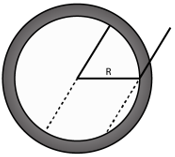I = mr2

About a point on a rim of the ring and the axis perpendicular to the plane of the ring, the moment of inertia = mR2 + mR2 = 2 mR2

=> mK2 = 2mR2

[Paralel axis theorem]

=> K = √(2R2) = 2R

Question 14: The radius of gyration of a uniform disc about a line perpendicular to the disc equals its radius. Find the distance of the line from the centre.

Solution:

Moment of inertia : I = mr2/2

Let us take a line parallel to this axis and at a distance d. Then the radius of gyration becomes r

Moment of inertia = I’ = L + md2

I’ = mr2/2 + md2

Also, I’ = mr2 (Given)

Equating above equations, we have

d2 = r2/2

or d = r/√2

Question 15: Find the moment of inertia of a uniform square plate of mass m and edge α about one of its diagonals.

Solution:

mass of that cross sectional area = m/a2 x (axdx)

moment of inertia along xx’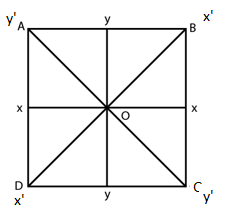Therefore, for two lines

I = 2m x a2/12

= ma2/6

Moment of inertia for pair of perpendicular diagonals:

I’ = 2m x a2/12 = ma2/6

Moment of inertia: I’ = 2I

[perpendicular axis theorem]

I = ma2/12

Question 16: The surface density (mass/area) of a circular disc of radius α depends on the distance from the centre as p(r) = A + Br. Find its moment of inertia about the line perpendicular to the plane of the disc through its centre.

Solution:

The inertia of the body for a point of mass is the product of the square of the radius with the mass of the body.

I = mAr

Where, I = moment of Inertia, A = area of the object and r = radius of the object.

The moment of inertia of a disc: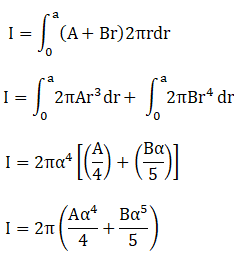Question 17: A particle of mass m is projected with a speed u at an angle θ with the horizontal. Find the torque of the weight of the particle about the point of projection when the particle is at the highest point.

Solution:

Formula used is that of a torque which tells us the mechanics of force which helps the object to rotate.

Torque = F. r …….(1)

F = force applied on the object and r = radius of the object turning.

The force of the object when in motion in linear path = τ = mgr/2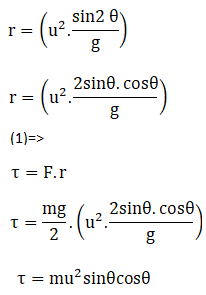Question 18: A simple pendulum of length l is pulled aside to make an angle θ with the vertical. Find the magnitude torque of the weight w of the bob about the point of suspension. When is the torque zero?

Solution:

Torque = F.r

The pendulum bob rotates at a distance of “l” from the center which is also the radius in terms of torque, the force, F = W

If angle of turning is θ, the radius in terms of turning angle is r = l sin θ

Torque = F.I sin θ = W. I sinθ

At lowest point the θ = 0, turning the torque equal to zero at the lowest point.

Question 19: When a force of 6.0 N is exerted at 30o to a wrench at a distance of 8 cm from the nut, it is just able to loosen the nut. What force F would be sufficient to loosen it if it acts perpendicularly to the wrench at 16 cm from the nut?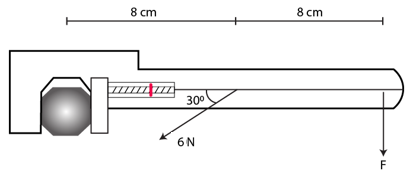Solution:

The force exerted into the wrench is F= 6N, the angle of motion is 30o. The distance from the nut to the wrench end is 16 cm.

Therefore, total torque acting at A about the point 0.

Torque = F.r sinθ = 6 x 0.08 x sin 30 o = 6 x 0.08 x (1/2)

Torque acting on the point B

6 x 0.08 x (1/2) = F.r sinθ

6 x 0.08 x (1/2) = F x 0.16 sinθ

F = 1.5 N

Question 20: Calculate the total torque acting on the body shown in figure (below) about the point O.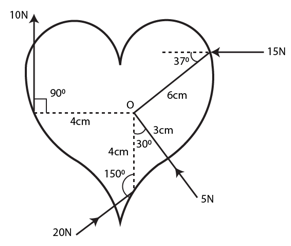Solution:

A torque which tells us the mechanics of force which helps the object to rotate.

Torque = F r sinθ

The torque acting on the point O due to the force of 15N:

τ15 = 15 x 6 x 10-2 sin 37o = 0.54 Nm

The torque acting on the point O due to the force of 10N:

τ10 = 10 x 4 x 10-2 = 0.40 Nm

The torque acting on the point O due to the force of 20N

τ20 = 20 x 4 x 10-2 sin 30o = 0.40 Nm

Due to torque negation between τ10 and τ20 which leaves them to zero, leaving the resultant torque equivalent to 0.54 Nm.

Question 21: A cubical block of mass m and edge α slides down a rough inclined plane of inclination θ with a uniform speed. Find the torque of the normal force acting on the block about its centre.

Solution:

a torque which tells us the mechanics of force which helps the object to rotate.

Torque = F r sinθ

The block of mass “m” moves with a uniform velocity on an inclined plane of angle θ, the force applied on the block.

F = mg sinθ

For the block not to roll the sum of the product of torque and force applied downwards and reactionary force due to the mass of the block should be zero.

τF + τFN = 0

F(a/2) = -FN

or – FN = (a/2) mg sinθ

Torque on the sliding object is (-a/2) mg sinθ

Question 22: A rod of mass m and length L, lying horizontally, is free to rotate about a vertical axis through its centre, A horizontal force of constant magnitude F acts on the rod at a distance of L/4 from the centre. The force is always perpendicular to the rod. Find the angle rotated by the rod during the time t after the motion starts.

Solution:

A torque which tells us the mechanics of force which helps the object to rotate.

Torque = Fr sinθ and I = mL2/12

The mass of rod is given as “m” and length “L”. So the torque acting on the rod:

τ = F x L/4

And, The moment of Inertia

I = mL2/12

The angle of rotation in terms of angular acceleration:

α = τ/I

= 3F/mL

and angle of rotation in term of angular length is

θ = ut + (1/2)αt2

Substituting the value of α and u = 0.

=> θ = (1/2)(3F/mL) t2

Question 23: A square plate of mass 120 g and edge 5.0 cm rotates about one of the edges. If it has a uniform angular acceleration of 0.2 rad/s2, what torque acts on the plate?

Solution:

The mass of the plate is 120g, the edges of the square is 5.0 cm and the angular acceleration is 0.2 rad/sec2

A torque which tells us the mechanics of force which helps the object to rotate.

Torque = Fr sinθ and I = mL2/12

The moment of Inertia of the plate: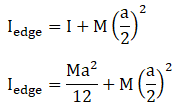By substituting the values of variables of mass and edge length

=> I = (0.12×0.05×0.05)/3

Torque produced by the plate: T = Iα

T = 0.0001 x 0.2 = 2 x 10-5 Nm

Question 24: Calculate the torque on the square plate of the previous problem if it rotates about a diagonal with the same angular acceleration.

Solution: Moment of inertia of a square plate abut its diagonal is ma2/12

Where m = mass of square plate and a = edges of the square

Torque produced = (ma2/12) x α

= [120×10-3x52x10-4]/[12×0.2]

= 0.5 x 10-5 N-m

Question 25: A flywheel of moment of inertia 5.0 kg-m2 is rotated at a speed of 60 rad/s. Because of the friction at the axle, it comes to rest in 5.0 minutes. Find (a) the average torque of the friction, (b) the total work done by the friction and (c) the angular momentum of the wheel 1 minute before it stops rotating.

Solution:

A flywheel of moment of inertia 5.0 kg-m2 is rotated at a speed of 60 rad/s.

Average torque = Iα

Calculation of work done from the torque of the flywheel:

W = (1/2) Iω2

The angular momentum of the wheel : L = I ω

Now, let us calculate the angular acceleration:

(a)the average torque of the flywheel

average torque = Iα = -Nm

and work done by the torque of the flywheel

W = (1/2) Iω2

W = 9 KJ

(b) angular momentum of the wheel in a time span of 4 minutes

ω = ωo + αt

= 60 – 240/5 = 12 rad/s

So, angular momentum = L = Iω = 5×12 = 60 kg/m2

Question 26: Because of the friction between the water in oceans with the earth’s surface, the rotational kinetic energy of the earth is continuously decreasing. If the earth’s angular speed decreases by 0.0016 rad/day in 100 years, find the average torque of the friction on the earth. Radius of the earth is 6400 km and its mass 6.0 × 1024 kg.

Solution:

The earth’s angular speed decreases by 0.0016 rad/day in 100 years (Given)

and 1 year = 365 x 56400 sec

Torque produced by ocean water in decreasing earth’s angular velocity is

τ = Iα

=(2/5) mr2 x (ω – ωo)/t

= (2/5) x 6 x 1024 x 642 x 1010 x [0.0016/264002 x 100 x 365]

= 5.678 x 1020 N-m

Question 27: A wheel rotating at a speed of 600 rpm (revolutions per minute) about its axis is brought to rest by applying a constant torque for 10 seconds. Find the angular deceleration and the angular velocity 5 seconds after the application of the torque.

Solution:

The relationship of velocity and acceleration in basic kinematics.

ω = ωo + αt

The angular velocity is taken in terms of rad/sec which is 10 and the time taken to rotate is 10 sec

0 = 10 + 10α

=> α = -1 rev/s2

Angular deceleration after 5 seconds we get

ω dec = ω + αt

=> ω dec = 5 rev/s

Question 28 : A wheel of mass 10 kg and radius 20 cm is rotating at an angular speed of 100 rev/mm when the motor is turned oft Neglecting the friction at the axle, calculate the force that must be applied tangentially to the wheel to bring it to rest in 10 revolutions.

Solution:

A torque which tells us the mechanics of force which helps the object to rotate.

Torque = Fr sin θ and I = (1/2) Mr2

where, F = force applied on the object; r= radius of the object turning.

Let θ be the turning angle and I = moment of Inertia,

Also, L is the length at which the force is applied.

let us find the acceleration using below formula:

ω’ = ω2 – 2θα

[ω = 100 rev/min = (5/8) rev/s = 10π/3 rad/s ] [0 = 10 rev = 20π rad and r = 0.2 m ]

0 = (3.22) 2 – 2α(10)

Moment of inertia: I = (1/2) Mr2 = (1/2)(10)(0.2) 2 = 0.2 kg m2

Therefore, force applied to the wheel = T = Fa

=> T = Fr

or Iα = Fr

=> F = 2π/36 x (1/0.2)

F = 0.87 N

Question 29: A cylinder rotating at an angular speed of 50 rev/s is brought in contact with an identical stationary cylinder. Because of the kinetic friction, torques act on the two cylinders, accelerating the stationary one and decelerating the moving one. If the common magnitude of the acceleration and deceleration be one revolution per second square, how long will it take before the two cylinders have equal angular speed?

Solution:

A cylinder rotating at an angular speed of 50 rev/s is brought in contact with an identical stationary cylinder.

The wheel rotates at a speed angular speed of 50 rev/s, at constant torque both acting in positive and negative acceleration.

For the 1st cylinder = ω = 50-αt

=> t = (ω-50)/-1 …(1)

For the 2nd cylinder =ω = α2t

=> t = ω/α2 = ω/1 …(2)

From (1) and (2),

ω = 25 rev/s

and (2)=> t = 25/1 = 25 sec

Question 30: A body rotating at 20 rad/s is acted upon by a constant torque providing it a deceleration of 2 rad/s2. At what time will the body have kinetic energy same as the initial value if the torque continues to act?

Solution:

Initial angular velocity = 20 rad/s

=> t1 = ω11 = 20/2 = 10 sec

As, the time taken for the torque to be equal to the kinetic energy

Here initial angular velocity = angular velocity at that instant

Time require to come to that angular velocity, t2 = ω22 = 20/2 = 10 sec

Total time requires = t1 + t2 = 10 + 10 = 20 sec

Question 31: A light rod of length 1 m is pivoted at its centre and two masses of 5 kg and 2 kg are hung from the ends as shown in figure (below). Find the initial angular acceleration of the rod assuming that it was horizontal in the beginning.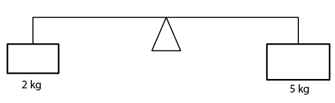Solution:

A light rod of length 1 m is pivoted at its centre and two masses of 5 kg and 2 kg are hung from the ends.

Here,

τ = Iα

Where τ = average frictional torque, I = moment of inertia and α =angular acceleration.

The torque produced on both the ends is:

5g x (l/2) – 2g x (l/2) = Iα

α = 6/7 g or 8.4 rad/s2

Question 32: Suppose the rod in the previous problem has a mass of 1 kg distributed uniformly over its length.

(a) Find the initial angular acceleration of the rod.

(b) Find the tension in the supports to the blocks of mass 2 kg and 5 kg.

Solution:

The given is that, the problem the ord has a mass 1 kg.

The angular acceleration is: τnet = Inet + α

The moment of the inertia = I = ml2/12

=> I = 1/12 kg m2

and total moment of inertia = I = 1.75 + 1/12 = 1.833 kg m2

(a) The angular acceleration of the rod at initial moment = α = T/I = 1.5 x (9.8/1.833) = 8 rad/s2

(b)

For 2 kg mass

T – 2g = 2a

T = 19.6 + 8 = 27.6 N

For 5 kg mass

T – 5g = 5a

= 49 – 20

= 29 N

Question 33: Figure (below) shows two blocks of masses m and M connected by a string passing over a pulley. The horizontal table over which the mass m slides is smooth. The pulley has a radius r and moment of inertia I about its axis and it can freely rotate about this axis. Find the acceleration of the mass M assuming that the string does not slip on the pulley.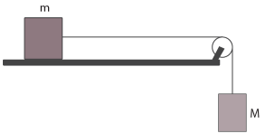Solution:

The blocks are of “m” and “M” masses, with radius of r pulley and moment of Inertia “I”

Using second law of Newton when the Force/Tension applied is equivalent to the product of mass and acceleration

F = ma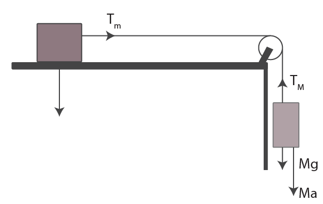Tension applied on the first block: T1 = M(g-a)

Tension applied on the 2nd block of mass: T2 = ma

Torque applied on the pulley : τ = Iα = Ia/r

Solving above equations, we get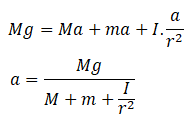Question 34: A string is wrapped on a wheel of moment of inertia 0.20 kg-m2 and radius 10 cm and goes through a light pulley to support a block of mass 2.0 kg as shown in figure (below). Find the acceleration of the block.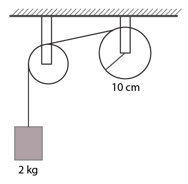Solution:

A string is wrapped on a wheel of moment of inertia = I = 0.20 kg-m2 and radius = r = 10 cm or 0.1 m.

Mass of the block = m = 2 kg

We know, mg – T = ma

and T = Ia/r2

From above equations,

mg = (m + I/r2)a

=> a = [2×9.8] / [2+0.2/0.01] = 0.89 m/s2, which is the acceleration of the block.

Question 35: Suppose the smaller pulley of the previous problem has its radius 5.0 cm and moment of inertia 0.10 kg-m2. Find the tension in the part of the string joining the pulleys.

Solution:

The moment of the inertia of the wheel, I = 0.20 and radius, r=10 cm and mass block of block, m = 2 kg. (Given)

We know, mg – T1 = ma ..(1)

(T1 – T2)r1 = I1 α ….(2)

T2 r2 = I2 α ….(3)

(2)=>

(T1 – I2 α/r1)r2 = I1 α

[Using value of T2]

=> T1 = [I1/r12 + I2/r22]a

on substituting T1 in (1)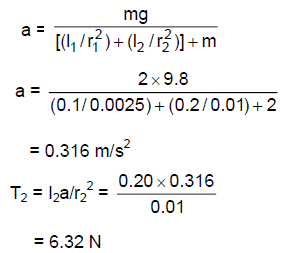Question 36: The pulleys in figure (below) are identical, each having a radius R and moment of inertia I. Find the acceleration of the block M.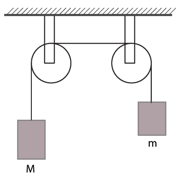Solution: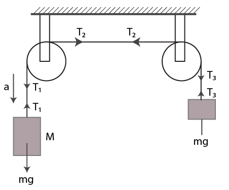From statement given,

Mg – T1 = Ma …(1)

(T2 – T1)R = la/R …(2)

(T2 – T3)R = la/R2 …(3)

and T3 – mg = ma ….(4)

From (2) and (3)

(T1 – T3) = 2la/R2 …..(5)

-mg + Mg + (T3 – T1) = Ma + ma ….(6)

Using equation (5), equation (6) implies

Mg – mg = Ma + ma + 2la/R2

or a = [(M-m)G]/[(M+m+2l/R2]

Question 37: The descending pulley shown in figure (below) has a radius 20 cm and moment of inertia 0.20 kg-m2. The fixed pulley is light and the horizontal plane frictionless. Find the acceleration of the block if its mass is 1.0 kg.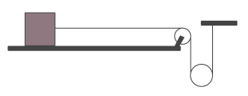Solution: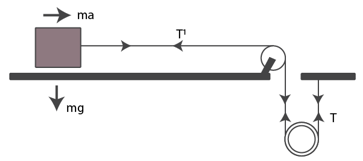Let T is the tension of plane and T’ of the side.

The angular acceleration of the pulley = α = (a/0.4) m/s2

So, the torque applied by the pulley due to the mass: I = (T – T’) x 0.20

= 0.20 kgm2

Again, α = T/I

=> (a/0.4) = (T – T’) x 0.20/0.20

=> (T – T’) = -25 a ….(1)

Find the value of mass M using the moment of Inertia

I = Mr2/2

or M = 10 kg

Now, Mg – T – T’ = Ma/2

Here M = 10 and g = 9.8

=> T + T’ = 98 – 5a …(2)

Adding (1) and (2) we get

2T = 98 – 5a – 2.5a

[Using formula, T = ma = 1xa = a]

2a = 98 – 5a – 2.5a

or a = 10.31 m/s2 .

Question 38: The pulley shown in figure (below) has a radius 10 cm and moment of inertia 0.5 kg-m2 about its axis. Assuming the inclined planes to be frictionless, calculate the acceleration of the 4.0 kg block.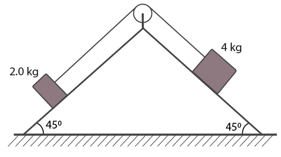Solution:

The radius of the pulley = 10 cm

Inertia = 0.5

Mass of the blocks are given as 2 kg and 4 kg.

The mass and tension relationship of the 4 kg block and the 2kg block :

m1g sinθ – T1 = m1 a . …..(1)

T1 – T2 = la/r^2 …(2)

T2 – m2 g sinθ = m2 a ….(3)

(1)+(3)=>

m1g sinθ – m2 g sinθ + (T2 – T1) = (m1 + m2)a

using equation (2) in above, we have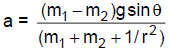=0.248 m/s2

Question 39: Solve the previous problem if the friction coefficient between the 2.0 kg block and the plane below it is 0.5 and the plane below the 4.0 kg block is frictionless.

Solution:

Form the above question’s figure,

m1g sinθ – T1 = m1a …(1)

T1 – T2 = la/r2 ….(2)

T2 – (m2 g sinθ + μ m2 g cosθ) = m2 a …(3)

(1)+(3)=>

m1g sinθ – (m2 g sinθ + μ m2 g cosθ) + (T2 -T1) = m1a + m2 a

Given, m1 = 4 kg, m2 = 2 kg, g = 9.8, sinθ = cosθ = 1/√2, μ = 0.5 and r = 0.1

Using (2), and substituting above values, we get

a = 0.125 m/s2

Question 40: A uniform meter stick of mass 200 g is suspended from the ceiling through two vertical strings of equal lengths fixed at the ends. A small object of mass 20 g is placed on the stick at a distance of 70 cm from the left end. Find the tensions in the two strings.

Solution:

Given, m1 = 200g, l = 1 m, m2 = 20g

The total tension formed due to the suspended weights: T1 + T2 = 2 + 0.2 = 2.2 …(1)

The rod is kept at an equilibrium when the rod is at rest

– T1 + T2 = 0.04/0.5 = 0.08 …(2)

Form (1) and (2)

T2 = 1.14 N

and (2)=> T1 = (1.14 – 0.08) = 1.06N

Question 41: A uniform ladder of length 10.0 m and mass 16.0 kg is resting against a vertical wall making an angle of 37o with it. The vertical wail is frictionless but the ground is rough. An electrician weighing 60.0 kg climbs up the ladder. If he stays on the ladder at a point 8.00 m from the lower end, what will be the normal force and the force of friction on the ladder by the ground? What should be the minimum coefficient of friction for the electrician to work safely?

Solution:

The length of the ladder = 10 m

Mass of the ladder is 16 kg which makes angle of 37 o and also given

Weight of the electrician is 60 kg, which stays at height of 8 cm.

Since the ladder should not slip or rotate, the torques expression is

mg(8 sin 37 o) + Mg(5 sin 37 o) = F2 (10 cos 37 o) = 0 and

60 x 9.8 x (8 sin 37 o) + 16 x 9.8 (5 sin 37 o) – F2 (10 cos 37 o) = 0

Where F1 and F2: forces of the masses in terms of reactionary force

=> F2 = 412N

let f be the friction force which is equal to the F2 due to equilibrium of the ladder

=> f = 412 N

Normal force = F1 = (m + M)g = (60+16)x 9.8 = 744.8 N

Now,

The minimum coefficient of friction = μ

=> μ = f/F1

[on substituting the values]

Question 42: Suppose the friction coefficient between the ground and the ladder of the previous problem is 0.540. Find the maximum weight of a mechanic who could go up and do the work from the same position of the ladder.

Solution:

The value of the reactionary force = R2 = 16g + mg and another value of R2 is R1

The relationship between R1 and R2 is

R1 x 10 cos 37o = 16g x 5 sin 37 o + mgx 60g x 8 x sin 37 o

The value of the reactionary forces = R1 is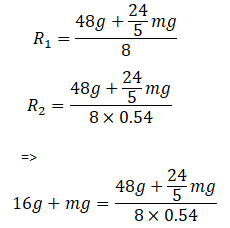Or m = 44 kg

Which is the mass of the mechanic that can go up the ladder.

Question 43: A 6.5 m long ladder rests against a vertical wall reaching a height of 6.0 m. A 60 kg man stands half way up the ladder. (a) Find the torque of the force exerted by the man on the ladder about the upper end of the ladder. (b) Assuming the weight of the ladder to be negligible as compared to the man and assuming the wall to be smooth, find the force exerted by the ground on the ladder.

Solution:

The length of the ladder = 6.5m

Weight of the man = 60kg and

(a) Torque is exerted at the upper end of the ladder and the there is no friction against the wall.

Torque due to weight of body

τ = Fr sinθ

τ = mg r sinθ

τ = 60x10x 6.5.2 sin√[1-(6/6.5)2] = 750 N

(b)

force exerted by the man through the ladder on the ground

F= mg = 60×10 = 600 N

Question 44: The door of an Amirah is 6 ft. high, 1.5 ft. wide and weighs 8 kg. The door is supported by two hinges situated at a distance of 1 ft. from the ends. If the magnitudes of the forces exerted by the hinges on the door are equal, find this magnitude.

Solution:

The force exerted by the two hinges: F1 + F2 = mg

or F1 + F2 = 8g

When F1 = F2

=> F1 = 40

[Using g = 10 m/s2]

The reactionary forces of the first hinge = M1 x 4 = 8g x 0.75

=> M1 = 15 N

the resultant force due to force and reactionary forces:

R = F12 + N12

= 402 + 152

or R = 43 N

Question 45: A uniform rod of length L rests against a smooth roller as shown in figure (below). Find the friction coefficient between the ground and the lower end if the minimum angle that the rod can make with the horizontal is θ.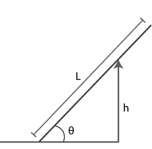Solution: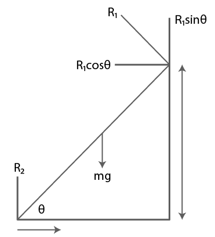The vertical and the horizontal component of the reactionary forces of the rod:

R2 = mg – R1 cosθ and R1 sinθ = μR2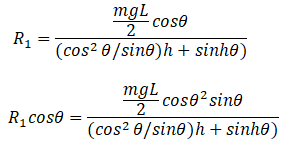The coefficient of friction: μ = [R1 sinθ]/R2

Using above equations, we get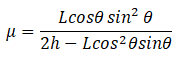Question 46: A uniform rod of mass 300 g and length 50 cm rotates at a uniform angular speed of 2 rad/s about an axis perpendicular to the rod through an end. Calculate (a) the angular momentum of the rod about the axis of rotation, (b) the speed of the centre of the rod and (c) its kinetic energy.

Solution:

(a) The average momentum

L = Iω

We are given, I = mr2/3

=> L = mr2/3 x ω

=> L = (0.3×0.52x2)/3

=> L = 0.05 kgm2/S

(b) Speed of the center of rod

v = ωr

=> v = 2 x (50/2) = 50 cm/s

(c) K.E. generated

K.E. = (1/2) x (0.0252 = 0.05 J

Question 47: A uniform square plate of mass 2.0 kg and edge 10 cm rotates about one of its diagonals under the action of a constant torque of 0.10 N-m. Calculate the angular momentum and the kinetic energy of the plate at the end of the fifth second after the start.

Solution:

Here (ma2/12) x α = 0.10 N – m

and ω = 60×5 = 300 rad/s

Therefore, average momentum = L = Iω

=> L = (0.10/60) x 300 = 0.5 kgm2/s

Then, the K.E. = (1/2) Iω2

= (0.10/60) x 3002

= 75 J

Question 48: Calculate the ratio of the angular momentum of the earth about its axis due to its spinning motion to that about the sun due to its orbital motion. Radius of the earth = 6400 km and radius of the orbit of the earth about the sun = 1.5 x 108 km.

Solution:

The angular momentum of earth about its axis:

(2/5) mR2 x (2π x 35400)

And the value of moment of inertia of earth: I = (2/5) MR2

Now the value of the sun’s average mass: mR(2π/86400 x 365)

Now, the ratio of the mass of sun and the mass of earth :